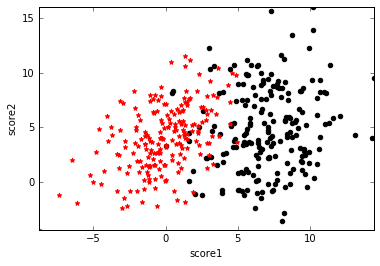# 初探逻辑回归1. $g(0)=0.5$，即在分类面上无法判断类标号是0还是1
2. $f(\mathbf{x})>0$时，$g(f(\mathbf{x}))>0.5$
3. $f(\mathbf{x})\rightarrow+\infty$$g(f(\mathbf{x}))\rightarrow 1$，且$g'(f(\mathbf{x}))\rightarrow 0$，即对于上右图右边的离群点，分类为1，且导数趋近于0，表示对其不敏感
4. $f(\mathbf{x})<0$时，$g(f(\mathbf{x}))<0.5$
5. $f(\mathbf{x})\rightarrow-\infty$$g(f(\mathbf{x}))\rightarrow 0$，且$g'(f(\mathbf{x}))\rightarrow 0$，和第3点类似$g(\mathbf{x})=\frac{1}{1+e^{-(\mathbf{w^Tx}+b)}}$

Sigmoid函数的另一个优点是，它把原始函数值映射到了概率空间，这样就可以用极大似然的方法求解参数$\mathbf{w}$$b$。下面用参数$\mathbf{\theta}$代表参数$\mathbf{w}$$b$，用$h_{\mathbf{\theta}}(\mathbf{x})$代表$g(f(\mathbf{x}))$。则有：

$P(y=1|\mathbf{x};\mathbf{\theta})=h_{\mathbf{\theta}}(\mathbf{x})$

$P(y=0|\mathbf{x};\mathbf{\theta})=1-h_{\mathbf{\theta}}(\mathbf{x})$

$P(y|\mathbf{x};\mathbf{\theta})=h_{\mathbf{\theta}}(\mathbf{x})^y(1-h_{\mathbf{\theta}}(\mathbf{x}))^{1-y}$

$L(\mathbf{\theta})=P(\mathbf{y}|X;\mathbf{\theta})=\prod\limits_{i}P(y^{(i)}|\mathbf{x}^{(i)};\mathbf{\theta})=\prod\limits_{i}h_{\mathbf{\theta}}(\mathbf{x}^{(i)})^{y^{(i)}}(1-h_{\mathbf{\theta}}(\mathbf{x}^{(i)}))^{1-y^{(i)}}$

$l(\mathbf{\theta})=logL(\mathbf{\theta})=\sum\limits_{i}y^{(i)}logh_{\mathbf{\theta}}(\mathbf{x}^{(i)})+(1-y^{(i)})log(1-h_{\mathbf{\theta}}(\mathbf{x}^{(i)}))$

$cost(h_{\theta}(\mathbf{x}),y) = \begin{cases}-log(h_{\theta}(\mathbf{x})) & \text {if y=1} \\ -log(1-h_{\theta}(\mathbf{x})) & \text{if y=0} \end{cases}$

$cost(h_{\theta}(\mathbf{x}),y) = -ylog(h_{\theta}(\mathbf{x})) - (1-y)log(1-h_{\theta}(\mathbf{x}))$

$J(\mathbf{\theta})=cost(h_{\theta}(X),\mathbf{y}) = \sum\limits_{i}-y^{(i)}logh_{\mathbf{\theta}}(\mathbf{x}^{(i)})-(1-y^{(i)})log(1-h_{\mathbf{\theta}}(\mathbf{x}^{(i)}))$

Softmax回归的损失函数如下，其实就是logistic回归损失函数的推广：## 1 thought on “初探逻辑回归”

This site uses Akismet to reduce spam. Learn how your comment data is processed.